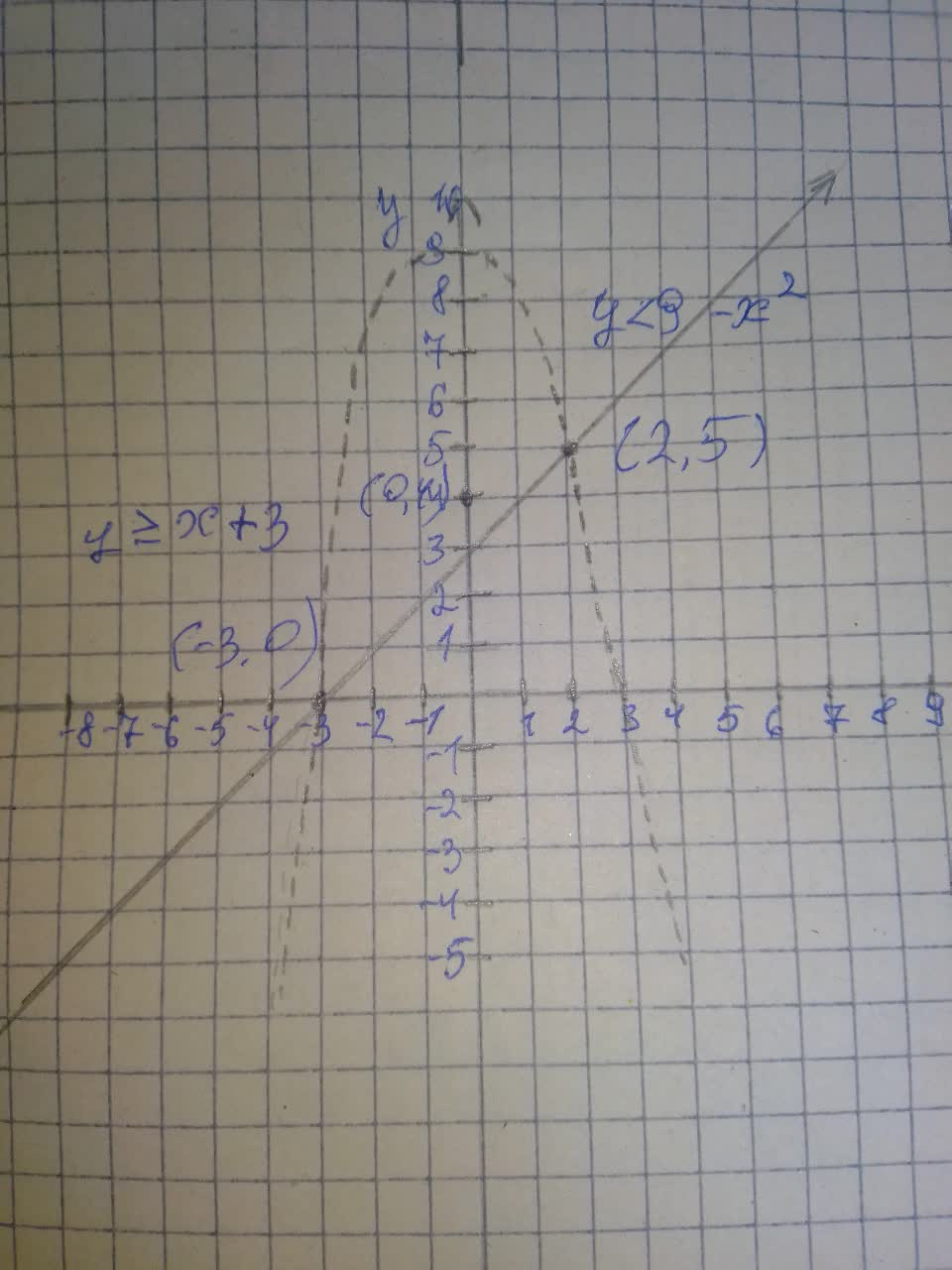Question# Graph the solution set of the system if inequalities \begin{cases}y < 9 - x^{2}\\y \geq x + 3 \end{cases}

Alternate coordinate systems
ANSWEREDSystems of Inequalities Graph the solution set of the system if inequalities. Find the coordinates of all vertices, and determine whether the solution set is bounded.
$$\begin{cases}y < 9 - x^{2}\\y \geq x + 3 \end{cases}$$2021-08-05

To graph:
The given system of inequality. Also find the coordinates of all vertices, and check whether the solution set is bounded.
Graph:
The given system of the inequalities is,
$$\displaystyle{y}{<}{9}-{x}^{{{2}}}\ldots..{\left({1}\right)}$$
$$\displaystyle{y}\geq{x}+{3}\ldots..{\left({2}\right)}$$
The corresponding equation of the inequality (1) is,
$$\displaystyle{y}={9}-{x}^{{{2}}}$$
Since, the inequality $$\displaystyle{y}{<}{9}-{x}^{{{2}}}$$ involves the strictly less than sign, then the points on the parabola $$\displaystyle{y}={9}-{x}^{{{2}}}$$ does not satisfy the inequality $$\displaystyle{y}{<}{9}-{x}^{{{2}}}$$.
Therefore, the parabola $$\displaystyle{y}={9}-{x}^{{{2}}}$$ itself is not the part of the solution set.
The corresponding equation of the inequality (2) is,
$$\displaystyle{y}={x}+{3}$$
Since, the inequality $$\displaystyle{y}\geq{x}+{3}$$ involves the greater than or equal to sign, then the points on the line $$\displaystyle{y}={x}+{3}$$ satisfies the inequality $$\displaystyle{y}\geq{x}+{3}$$
Therefore, the line $$\displaystyle{y}={x}+{3}$$ itself is the part of the solution set.
Consider the test points (0,4) to check whether the solution satisfies each inequality of the given system.
Substitute 0 for x and 4 for y in the inequality $$\displaystyle{y}{<}{9}-{x}^{{{2}}}$$
$$\displaystyle{y}{<}{9}-{x}^{{{2}}}$$
$$\displaystyle{4}{<}{9}-{0}$$
$$\displaystyle{4}{<}{9}$$
The point (0,4) is inside the parabola $$\displaystyle{y}={9}-{x}^{{{2}}}$$ and satisfies the inequality $$\displaystyle{y}{<}{9}-{x}^{{{2}}}$$. So it is the part of the solution set.
Substitute 0 for x and 4 for y in the inequality $$\displaystyle{y}\geq{x}+{3}$$.
$$\displaystyle{y}\geq{x}+{3}$$
$$\displaystyle{4}\geq{0}+{3}$$
$$\displaystyle{4}\geq{3}$$
The points (0,4) is below the line $$\displaystyle{y}={x}+{3}$$ and satisfies the inequality $$\displaystyle{y}\geq{x}+{3}$$. So it is the part of the solution set.
Therefore, the test point(0,4) satisfies each inequality of the given system.
The solution set of the given system of inequalities is the intersection of the solutions of each of the given inequality.
Therefore, the solution set is shown as shaded region.The vertices occur at the points of intersection of the corresponding equation of the given system of inequalities.
$$\displaystyle{y}={9}-{x}^{{{2}}}\ldots..{\left({3}\right)}$$
$$\displaystyle{y}={x}+{3}\ldots..{\left({4}\right)}$$
It is observed from Figure 1 that the parabola $$\displaystyle{y}={9}-{x}^{{{2}}}$$ and line $$\displaystyle{y}={x}+{3}$$ intersect each other.
Substitute $$\displaystyle{x}+{3}$$ for y in equation (3).
$$\displaystyle{y}={9}-{x}^{{{2}}}$$
$$\displaystyle{x}+{3}={9}-{x}^{{{2}}}$$
$$\displaystyle{x}^{{{2}}}+{x}={9}-{3}$$
$$\displaystyle{x}^{{{2}}}+{x}-{6}={0}$$
Further solve the above equation for the value of x.
$$\displaystyle{x}^{{{2}}}+{3}{x}-{2}{x}-{6}={0}$$
$$\displaystyle{\left({x}-{2}\right)}{\left({x}+{3}\right)}={0}$$
$$\displaystyle{x}+{3}={0}$$ or $$\displaystyle{x}-{2}={0}$$
$$\displaystyle{x}=-{3}$$ or $$\displaystyle{x}={2}$$
Therefore, the x-coordinate of vertex are -3 and 2.
Substitute -3 for x in equation (3).
$$\displaystyle{y}={9}-{x}^{{{2}}}$$
$$\displaystyle{y}={9}-{\left(-{3}\right)}^{{{2}}}$$
$$\displaystyle{y}={0}$$
Substitute 2 for x in exuation (3).
$$\displaystyle{y}={9}-{x}^{{{2}}}$$
$$\displaystyle{y}={9}-{\left({2}\right)}^{{{2}}}$$
$$\displaystyle{y}={9}-{4}$$
$$\displaystyle{y}={5}$$
Therefore, the y-coordinate of vertex are 0 and 5.
Therefore, the vertices of the shaded region are (-3,0) and (2,5).
It is observed from Figure 1 that the shaded region is enclosed by the boundary lines of the given system of inequalities.
Therefore, the shaded region is bounded.
Interpretation:
The solution set of the given system of inequality lies in 1 and 2 quadrant as shown in Figure 1.
Conclusion:
Thus, the vertices of the given system of inequalities are (-3,0) and (2,5), the solution set is bounded.